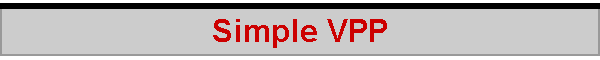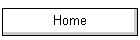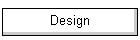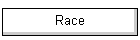I've developed the "acceleration" spreadsheet from the Acceleration page into a simple VPP spreadsheet (approx 85 kb) to play with.  The basic operation of the spreadsheet is similar, and is described there.  This page looks at the polar diagram and other features added.

Please note that the spreadsheet uses two simple macros to populate two data tables.  If you have your Excel software set to query you if it finds macros in the spreadsheet it is loading, this is the reason.

Update November 2020.  David Kender has kindly provided an updated spreadsheet with macros that work with more recent versions of Excel.

The VPP spreadsheet calculates sail drive depending upon the boat's heading.  This is done rather crudely, of course.  The major point to note is that, when the sheeting angle reaches 90 degrees, the spreadsheet starts to use the Cd from the running scenario in combination with the Cl from the beating and reaching scenario.  There is a little "jump" in calculated values when this happens, and the resulting graph lines may have an unwelcome wiggle.

### Sheeting angle

For interest, given a heading and a wind, the spreadsheet calculates the sheeting angle of the sails (well, the jib, really) that is assumed to be appropriate, given the angle of attack used to extract maximum lift.  The default angle of attack is set to 15 degrees in the spreadsheet, but you can change this if you prefer to 18 or 20 or whatever.  For example, the spreadsheet suggests a sheeting angle of about 15 degrees for maximum speed when the heading is 40 degrees in a wind of 2.5.  Not too far away from reality.  You can play with different wind speeds to see how the suggested sheeting angle changes.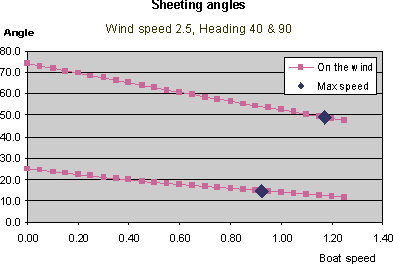The interesting thing about the graph of sheeting angles, such as those shown here, is the point it makes about an appropriate sheeting angle when the boat has not reached maximum speed or is starting from rest -- sheet out, and gradually sheet in as maximum speed is attained.  And the spreadsheet gives you a hint about the time over which you should be gradually sheeting in.  For the example of a wind speed of 2.5 and a heading of 40, this turns out to be over a period of about 3 seconds.

The graph also shows sheeting angles for reaching, in this case when on a heading of 90 degrees -- that is, the true wind is exactly on the beam.  Because of the angle of the apparent wind, you can see that the suggested sheeting angle here is about 50 degrees when at maximum speed, starting from 75 when the boat is at rest, for a wind speed of 2.5.  Again, the spreadsheet gives a hint that you should sheet in as the boat gains speed over a period of about 1.8 seconds if starting from rest with a wind speed of 2.5, as in this case.

The second sheeting graph illustrates the spreadsheet results when the wind speed is low, at 0.50.  Notice that, while the suggested sheeting angle of 15 degrees seems the same as for the higher wind speed, the boat is at a heading of 45 degrees -- that is, footing.

Look at the result for reaching -- the ideal sheeting angle is around 40 degrees at maximum speed.  What the spreadsheet is showing is that, at lower wind speeds, the 'apparent wind effect' is even more marked.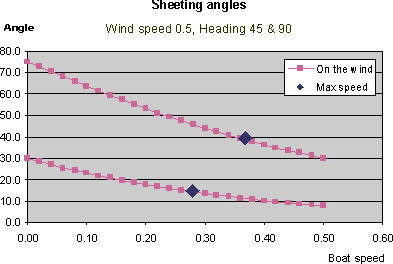Finally, at this low wind speed, have a look at the spreadsheet results for how long it takes to get anywhere near maximum speed -- somewhere between 15 to 20 seconds beating, 14 to 18 seconds reaching!  Of course, we know that the values here are grossly approximate because we don't have a calibrated spreadsheet, but the point being made is valid enough -- it takes a long time to get up to speed in light airs, so take your time about sheeting in while the boat does so.

### Polar diagram

This is really why the VPP spreadsheet might be interesting.  Pressing <CTRL>-<p> or clicking on the 'Polar' button starts the "Polar" macro, at the bottom of the spreadsheet (scroll down!).  This yields a polar diagram, similar to the one below, for a given wind speed.  Remember to set the desired wind speed into the spreadsheet first.  The macro then populates a couple of rows of data by repeatedly running the spreadsheet for a variety of headings.The polar diagram here has the results for three different wind speeds superimposed.  The red line is for 2.5, the green for 1.5, and the blue for 0.5.  According to the spreadsheet, we see the classic lobes showing maximum speed while reaching, but these lobes do not extend into the broad reaching area -- possibly because we are not modelling running spinnakers or asymmetrical spinnakers.

The polar also shows that, relatively speaking, the reaching lobes are less accentuated at higher wind speeds, and more accentuated for lighter winds.  That is, there is more of a difference between maximum speeds reaching versus running when the wind is light.  So if the mark is not directly downwind, but at a bearing of, say, 135, it might well be better to "tack" downwind when the wind is light.  The polar below shows the result for light airs at a larger scale, and the relatively larger lobes are more apparent.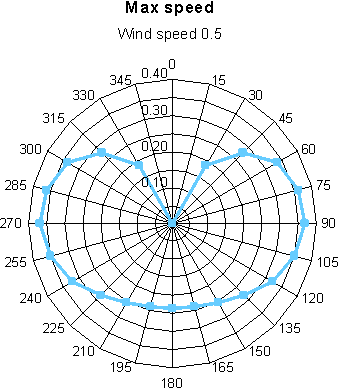### VMG

The spreadsheet calculates the VMG, the speed of the boat directly to windward.  Playing with headings around 40 or 45, and with various wind speeds, the VPP predicts that maximum VMG is obtained if you foot somewhat in light airs, and pinch in heavy air.  Using the Solver function, a heading of about 46 degrees gives maximum VMG for a wind speed of 0.25, and this drops to 39 degrees when over-canvassed with a wind speed of 3.0.

### "Max" macro

A second macro is provided which populates a data table of maximum boat speeds and time to maximum speeds, for a set heading, given a variety of wind speeds.  The resulting graphs are discussed on the Acceleration page.  Press <CTRL>-<m>, or click on the 'Max' button to activate this macro.

### Some notes on using the VPP spreadsheet

The "On the wind" calculations are made for a specified heading, entered in degrees.  The apparent wind speed and direction are then calculated.  In order to obtain the forward drive force of the sails, the spreadsheet needs to know the angle of attack of the sails to the wind.  In the default spreadsheet, this is set at 15 degrees.  You can change this to any other values you wish.

To save some screen space, a couple of columns are sized too narrow to show their values.  They show "#" instead.  This isn't a problem.

In order to give outputs which are approximately (very approximately!) correct for an IOM, the drag and drive forces have been arbitrarily scaled.  The scale factors used (S scale, W scale, F scale, and T mult) give speeds in metres per second, time in seconds, and forces in grams.

To check that, overall, the maximum speed values are sensible, the spreadsheet has a calculation of the "hull speed", the theoretical maximum speed for a displacement yacht, calculated from its waterline length.  For the IOM, this is 1.23 m/s.  So I've used a set of scaling factors which gives maximum speed of 1.17 on a reach with a wind speed of 2.5 -- the top of the wind range for an IOM in No.1 rig.

The macros are very crude, and refer to particular cells in the spreadsheet.  If you add or delete rows or columns, you'll knock the macros out of whack...  Sorry.

2020-11-11